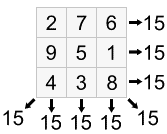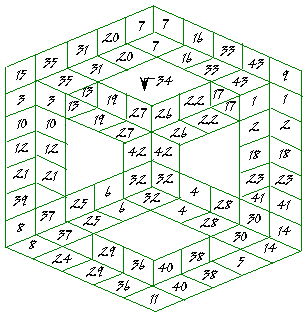# Impossible magic cube

Magic square is widely known mathematical figure with different numbers in it's cells, where numbers in all rows, all columns, and both diagonals sum to the same constant. You can see below a simple example of magic square.There are also magic cubes with the same summing properties. But also more complicated magic figures can be designed.

Dr. Clifford Pickover, in his excellent book on magic squares, illustrates impossible magic cube. The impossible cube uses the numbers 1 to 43 in 8 lines of 5, 2 lines of 7 and 1 line of 3. The sum is 108. Note that one cube (number 34) is hidden.The model of impossible magic cube was found in the article Unusual magic cubes by Harvey Heinz.